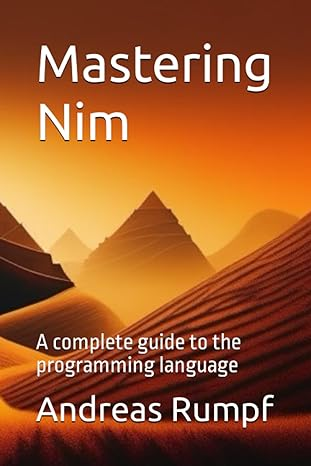# Efficient, expressive, elegant

## Nim is a statically typed compiled systems programming language. It combines successful concepts from mature languages like Python, Ada and Modula.

### Efficient

• Nim generates native dependency-free executables, not dependent on a virtual machine, which are small and allow easy redistribution.
• The Nim compiler and the generated executables support all major platforms like Windows, Linux, BSD and macOS.
• Nim's memory management is deterministic and customizable with destructors and move semantics, inspired by C++ and Rust. It is well-suited for embedded, hard-realtime systems.
• Modern concepts like zero-overhead iterators and compile-time evaluation of user-defined functions, in combination with the preference of value-based datatypes allocated on the stack, lead to extremely performant code.
• Support for various backends: it compiles to C, C++ or JavaScript so that Nim can be used for all backend and frontend needs.

### Expressive

• Nim is self-contained: the compiler and the standard library are implemented in Nim.
• Nim has a powerful macro system which allows direct manipulation of the AST, offering nearly unlimited opportunities.

### Elegant

• Macros cannot change Nim's syntax because there is no need for it — the syntax is flexible enough.
• Modern type system with local type inference, tuples, generics and sum types.
• Statements are grouped by indentation but can span multiple lines.
``` import std/strformat type Person = object name: string age: Natural # Ensures the age is positive let people = [ Person(name: "John", age: 45), Person(name: "Kate", age: 30) ] for person in people: # Type-safe string interpolation, # evaluated at compile time. echo(fmt"{person.name} is {person.age} years old") # Thanks to Nim's 'iterator' and 'yield' constructs, # iterators are as easy to write as ordinary # functions. They are compiled to inline loops. iterator oddNumbers[Idx, T](a: array[Idx, T]): T = for x in a: if x mod 2 == 1: yield x for odd in oddNumbers([3, 6, 9, 12, 15, 18]): echo odd # Use Nim's macro system to transform a dense # data-centric description of x86 instructions # into lookup tables that are used by # assemblers and JITs. import macros, strutils macro toLookupTable(data: static[string]): untyped = result = newTree(nnkBracket) for w in data.split(';'): result.add newLit(w) const data = "mov;btc;cli;xor" opcodes = toLookupTable(data) for o in opcodes: echo o ``` ``` var conditional = 42 if conditional < 0: echo "conditional < 0" elif conditional > 0: echo "conditional > 0" else: echo "conditional == 0" var ternary = if conditional == 42: true else: false var another = if conditional == 0: "zero" elif conditional mod 2 == 0: "even" else: "odd" # Case switch. var letter = 'c' case letter of 'a': echo "letter is 'a'" of 'b', 'c': echo "letter is 'b' or 'c'" of 'd'..'h': echo "letter is between 'd' and 'h'" else: echo "letter is another character" ``` ``` import std/math # Basic math. assert 1 + 2 == 3 # Sum assert 4 - 1 == 3 # Subtraction assert 2 * 2 == 4 # Multiplication assert 4 / 2 == 2.0 # Division assert 4 div 2 == 2 # Integer Division assert 2 ^ 3 == 8 # Power assert 4 mod 2 == 0 # Modulo assert (2 xor 4) == 6 # XOR assert (4 shr 2) == 1 # Shift Right assert PI * 2 == TAU # PI and TAU assert sqrt(4.0) == 2.0 # Square Root assert round(3.5) == 4.0 # Round assert isPowerOfTwo(16) # Powers of Two assert floor(2.9) == 2.0 # Floor assert ceil(2.9) == 3.0 # Ceil assert cos(TAU) == 1.0 # Cosine assert gcd(12, 8) == 4 # Greatest common divisor assert trunc(1.75) == 1.0 # Truncate assert floorMod(8, 3) == 2 # Floor Modulo assert floorDiv(8, 3) == 2 # Floor Division assert hypot(4.0, 3.0) == 5.0 # Hypotenuse assert gamma(4.0) == 6.0 # Gamma function assert radToDeg(TAU) == 360.0 # Radians to Degrees assert clamp(1.4, 0.0 .. 1.0) == 1.0 # Clamp assert almostEqual(PI, 3.14159265358979) assert euclDiv(-13, -3) == 5 # Euclidean Division assert euclMod(-13, 3) == 2 # Euclidean Modulo ``` ``` import std/[strutils, strscans] assert "con" & "cat" == "concat" assert " a ".strip == "a" assert "42".parseInt == 42 assert "3.14".parseFloat == 3.14 assert "0x666".parseHexInt == 1638 assert "TrUe".parseBool == true assert "0o777".parseOctInt == 511 assert "a".repeat(9) == "aaaaaaaaa" assert "abc".startsWith("ab") assert "abc".endsWith("bc") assert ["a", "b", "c"].join == "abc" assert "abcd".find("c") == 2 assert "a x a y a z".count("a") == 3 assert "A__B__C".normalize == "abc" assert "a,b".split(",") == @["a", "b"] assert "a".center(5) == " a " assert "a".indent(4) == " a" assert " a".unindent(4) == "a" for word in tokenize("This is an example"): echo word let (ok, year, month, day) = scanTuple("1000-01-01", "\$i-\$i-\$i") if ok: assert year == 1000 assert month == 1 assert day == 1 ``` ``` import std/[sugar, tables, sets, sequtils, strutils] let variable0 = collect(newSeq): for item in @[-9, 1, 42, 0, -1, 9]: item * 2 assert variable0 == @[-18, 2, 84, 0, -2, 18] let variable1 = collect(initTable): for key, value in @[0, 5, 9]: {key: value div 2} assert variable1 == {0: 0, 1: 2, 2: 4}.toTable let variable2 = collect(initHashSet): for item in @[-9, 1, 42, 0, -1, 9]: {item + item} assert variable2 == [2, 18, 84, 0, -18, -2].toHashSet assert toSeq(1..15).mapIt( if it mod 15 == 0: "FizzBuzz" elif it mod 5 == 0: "Buzz" elif it mod 3 == 0: "Fizz" else: \$it ).join(" ").strip == "1 2 Fizz 4 Buzz Fizz 7 8 Fizz Buzz 11 Fizz 13 14 FizzBuzz" ```

# Recent articles

## Mastering Nim, 2nd edition

Discover the secret of Nim!

## This Month with Nim: July and August 2023

Webdev, webdev, webdev, and a backup program!# Community

## Forum

forum.nim-lang.org

r/nim

StackOverflow

nim-lang/Nim